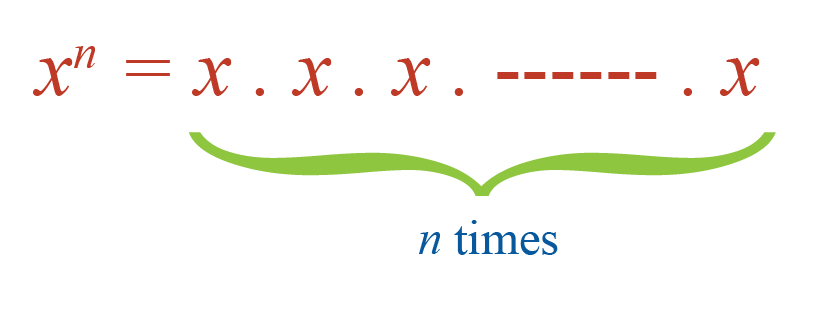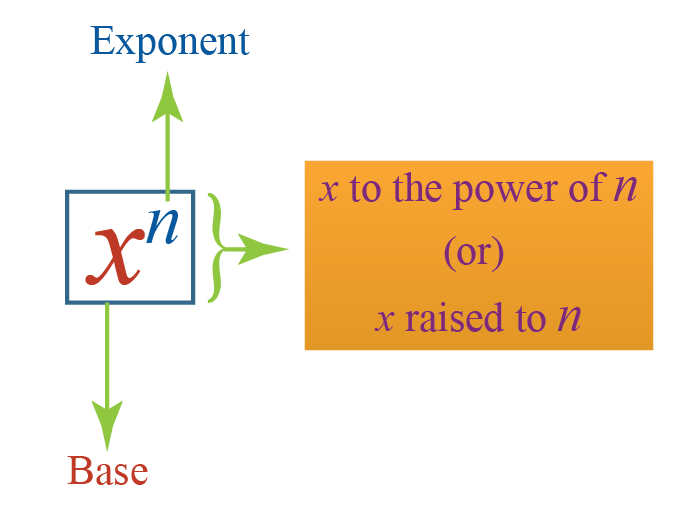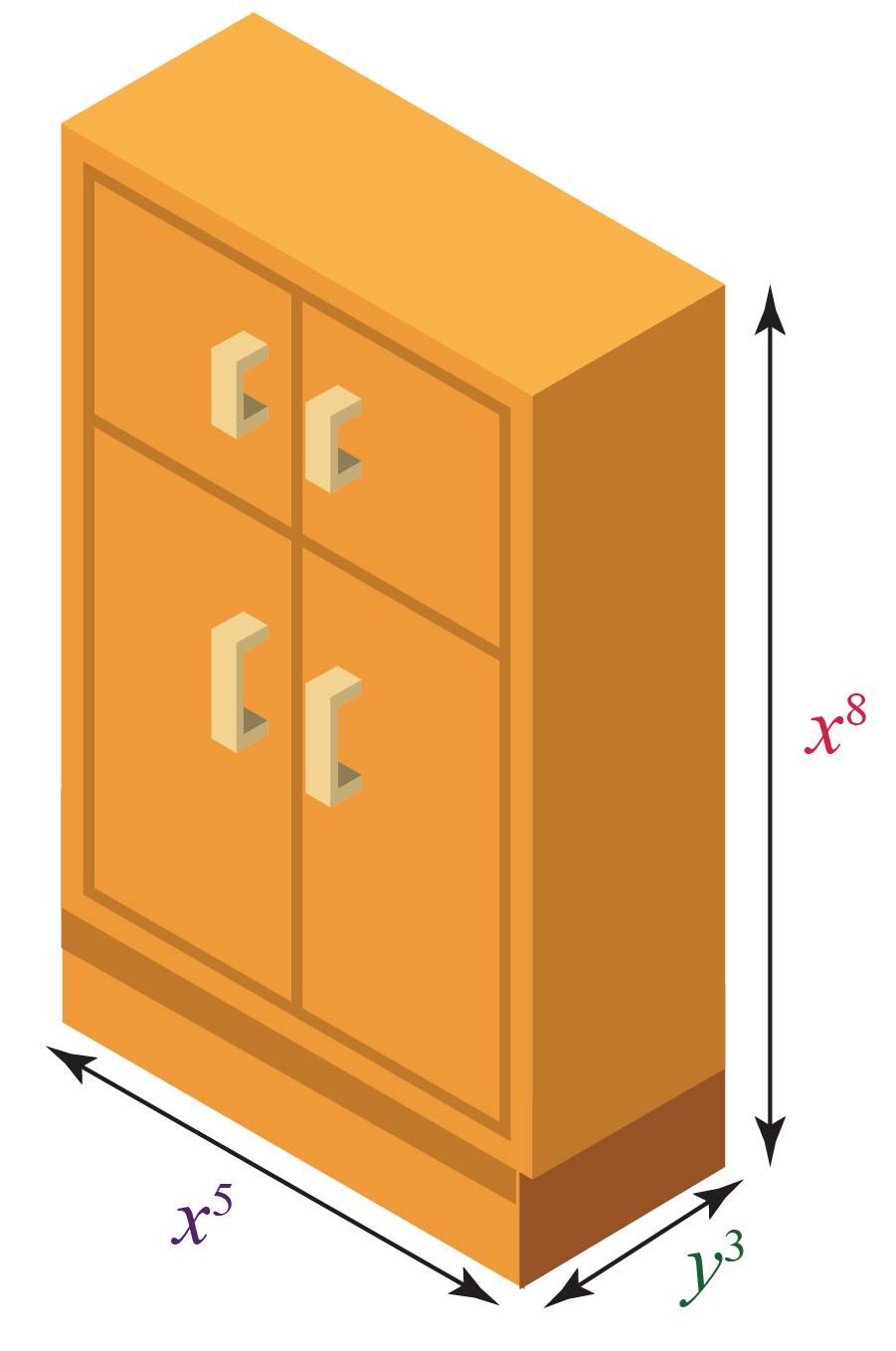# Exponents

 1 Definition of Exponents 2 Exponents Calculator 3 Laws (Properties or Rules) of Exponents 4 Important Notes on Exponents 5 Solved Examples on Exponents 6 Practice Questions on Exponents 7 Tips and Tricks 8 FREE Exponents Worksheets 9 Maths Olympiad Sample Papers 10 Frequently Asked Questions (FAQs)

We at Cuemath believe that Math is a life skill. Our Math Experts focus on the “Why” behind the “What.” Students can explore from a huge range of interactive worksheets, visuals, simulations, practice tests, and more to understand a concept in depth.

Book a FREE trial class today! and experience Cuemath's LIVE Online Class with your child.

## Definition of Exponents

The exponent of a number shows how many times the number is multiplied by itself.

For example, $$2 \times 2 \times 2 \times 2$$ can be written as $$2^4$$; as $$2$$ is multiplied by itself $$4$$ times.

Here, $$2$$ is called the "base" and $$4$$ is called the "exponent" or "power."

In general, $$x^n$$ means that $$x$$ is multiplied by itself for $$n$$ times.Here,

• $$x$$ is called the "base"
• $$n$$ is called the "exponent" or "power"
• $$x^n$$ is read as "$$x$$ to the power of $$n$$" (or) "$$x$$ raised to $$n$$"### Examples of Exponents

Some examples of exponents are as follows:

• \begin{align} 3 \times 3 \times 3 \times 3 \times 3 &= 3^5\end{align}

• \begin{align}-2 \times -2 \times -2 &= (-2)^3\end{align}

• \begin{align}a \times a \times a \times a \times a \times a &=a^6\end{align}

### Why are Exponents Important?

Exponents are important because, without them, the products where a number is repeated by itself for many times is very difficult to write.

For example, it is very easy to write $$5^7$$ instead of writing $$5 \times 5 \times 5 \times 5 \times 5 \times 5 \times 5$$.

## Exponents Calculator

Here is the "Exponents Calculator".

Here, you can set the values of the base and the exponent.

Then it will show the corresponding value.

## Laws (Properties or Rules) of Exponents

The laws (properties or rules) of exponents are used to solve problems involving exponents.

The laws of exponents are mentioned below.

For each law, an example is provided to see the difference in solving equations when we use laws of exponents versus when we do not use the laws.

Law of Product:

$$\mathbf{a^m \times a^n = a^{m + n}}$$

Using the law

Without using the law
\begin{align}2^3 \times 2^5 &= 2^{3+5}\\&=2^8 \end{align} \begin{aligned} 2^{3} \times 2^{5} &=(2 \cdot 2 \cdot 2)(2 \cdot 2 \cdot 2 \cdot 2) \\ &=2^{8} \end{aligned}

Law of Quotient:

$$\mathbf{\dfrac{a^m}{a^n}= a^{m-n}}$$

Using the law

Without using the law
\begin{align}\dfrac{2^5}{2^3}&= 2^{5-3}\\&=2^2\end{align} \begin{aligned} \frac{2^{5}}{2^{3}} &=\frac{2 \cdot 2 \cdot 2 \cdot 2}{2 \cdot 2 \cdot 2} \\ &=2^{2} \end{aligned}

Law of Zero Exponent:

$$\mathbf{a^0=1}$$

Using the law

Without using the law
$$2^0 =1$$

\begin{aligned}2^{0} &=2^{5-5} \\&=\frac{2^{5}}{2^{5}}\left[\because \frac{a^{m}}{a^{n}}=a^{m-n}\right] \\&=\frac{2 \cdot 2 \cdot 2 \cdot 2 \cdot 2}{2 \cdot 2 \cdot 2 \cdot 2 \cdot 2} \\&=1\end{aligned}

Law of Negative Exponent:

$$\mathbf{a^{-m} = \dfrac{1}{a^m}}$$

Using the law

Without using the law

$$2^{-2} = \dfrac{1}{2^2}$$

\begin{aligned} 2^{-2} &=2^{0-2} \\ &=\frac{2^{0}}{2^{2}}\left[\because \frac{a^{m}}{a^{n}}=a^{m-n}\right] \\ &=\frac{1}{2^{2}}\left[\because 2^{0}=1\right] \end{aligned}

Law of Power of a Power:

$$\mathbf{(a^m)^n=a^{mn}}$$

Using the law

Without using the law

$$(2^2)^3 = 2^6$$

\begin{aligned}
\left(2^{2}\right)^{3} &=\left(2^{2}\right)\left(2^{2}\right)\left(2^{2}\right) \\
&=(2 \cdot 2)(2 \cdot 2)(2 \cdot 2) \\
&=2^{6}
\end{aligned}

Law of Power of a Product:

$$\mathbf{(ab)^m=a^mb^m}$$

Using the law

Without using the law
$$(xy)^3 = x^3y^3$$ \begin{aligned} (x y)^{3} &=(x y)(x y)(x y) \\ &=(x x x)(y y y) \\ &=x^{3} y^{3} \end{aligned}

Law of Power of a Quotient:

$$\mathbf{\left(\dfrac{a}{b}\right)^m= \dfrac{a^m}{b^m}}$$

Using the law

Without using the law
$$\left(\dfrac{x}{y}\right)^3= \dfrac{x^3}{y^3}$$ \begin{aligned} \left(\frac{x}{y}\right)^{3} &=\frac{x}{y} \cdot \frac{x}{y} \cdot \frac{x}{y} \\ &=\frac{x^{3}}{y^{3}} \end{aligned}

From these tables above, you would have got an idea of how and why a particular law works and how these laws of exponents make our work easier.

Let's try and solve a few examples using these laws.

Help your child score higher with Cuemath’s proprietary FREE Diagnostic Test. Get access to detailed reports, customized learning plans, and a FREE counseling session. Attempt the test now.

## Solved Examples

 Example 1

The dimensions of a wardrobe are $$x^5$$ cm, $$y^3$$ cm and $$x^8$$ cm. Find its volume.Solution:

The dimensions of the wardrobe are:

\begin{aligned} \text{Length, } l &= x^5 \text{ cm}\\[0.2cm] \text{Width, } w &= y^3 \text{ cm}\\[0.2cm] \text{Height, } h &= x^8 \text{ cm} \end{aligned}

The volume of the wardrobe, $$V$$ is:

\begin{aligned} V &= lwh\\[0.2cm]&=x^5 \cdot y^3 \cdot x^8\\[0.2cm]&=x^{13} \,y^3 \; [\because a^m \cdot a^n = a^{m+n} ] \end{aligned}

 $$\therefore V =x^{13} \,y^3$$
 Example 2

Evaluate the following expression as an integer:

$\left(\dfrac{1}{2}\right)^{-2}+\left(\dfrac{1}{3}\right)^{-2}+\left(\dfrac{1}{5}\right)^{-2}$

Solution:

We will simplify the given expression using the laws of exponents.

\begin{align} &= \frac{1^{-2}}{2^{-2}} + \frac{1^{-2}}{3^{-2}} + \frac{1^{-2}}{5^{-2}} \;[\because \left(\dfrac{a}{b}\right)^m\!= \dfrac{a^m}{b^m}] \\\\ &= \frac{1}{2^{-2}}+\frac{1}{3^{-2}}+\frac{1}{5^{-2}} \;[\because 1^{-2}=1] \\\\ &= 2^2+3^2+5^2 \; [\because \dfrac{1}{a^{-m}} = a^m]\\\\ &= 4+9+25\\\\ &=38 \end{align}

 $$\therefore$$ \begin{align}\left(\frac{1}{2}\right)^{-2}\!\!+\!\left(\frac{1}{3}\right)^{-2}\!\!+\!\left(\frac{1}{5}\right)^{-2} \!= 38\end{align}
 Example 3

Simplify the following expression:

$\dfrac{\left(x^{a+b}\right)^{2} \times\left(x^{b+c}\right)^{2} \times\left(x^{c+a}\right)^{2}}{\left(x^{a} \times x^{b} \times x^{c}\right)^{3}}$

Solution:

We will simplify the given expression using the laws of exponents.

\begin{align}
&\frac{\left(x^{a+b}\right)^{2} \times\left(x^{b+c}\right)^{2} \times\left(x^{c+a}\right)^{2}}{\left(x^{a} \cdot x^{b} \cdot x^{c}\right)^{3}}\\\\
&= \frac{x^{2a+2b}\times x^{2b+2c}\times x^{2c+2a}}{x^{3a} \times x^{3b} \times x^{3c}} \;[\because \! (a^m)^n \!\!=\! a^{mn}; (ab)^m \!\!=\! a^m b^m ]\\\\ &=\frac{x^{2a+2b+2b+2c+2c+2a}}{x^{3a+3b+3c}} \;[\because \!a^m \!\!\times\! a^n \!\!=\!a^{m+n} ]\\\\ &= \frac{x^{4a+4b+4c}}{x^{3a+3b+3c}}\\\\ &=x^{4a+4b+4c - (3a+3b+3c)} \; [\because \!\dfrac{a^m}{a^n}\!\!=\! a^{m-n} ]\\\\&= x^{a+b+c}
\end{align}

 \begin{align}\therefore\!\frac{\left(x^{a+b}\right)^{2} \!\times\!\left(x^{b+c}\right)^{2} \!\times\!\left(x^{c+a}\right)^{2}}{\left(x^{a} \!\cdot\! x^{b} \!\cdot\! x^{c}\right)^{3}} \!= x^{a+b+c}\end{align}
 Example 4

In a forest, each tree has about $$5^7$$ leaves and there are about $$5^3$$ trees in the forest.

Find the total number of leaves.Solution:

The number of trees in the forest = $$5^3$$

The number of leaves in each tree = $$5^7$$

The total number of leaves are:

\begin{aligned} 5^3 \times 5^7 &= 5^{10} \; [\because a^m \times a^n = a^{m+n}] \end{aligned}

 $$\therefore$$ Total number of leaves $$=5^{10}$$
 Example 5

Find the value of $$x$$ if:

$\left(\frac{1}{7}\right)^{2} \times\left(\frac{1}{7}\right)^{-9}=\left(\frac{1}{7}\right)^{2 x-3}$

Solution:

The given equation is:

\begin{aligned} \left(\frac{1}{7}\right)^{2} \!\!\!\times\!\left(\frac{1}{7}\right)^{-9}&\!\!\!=\!\left(\frac{1}{7}\right)^{2 x-3} \\[0.3cm] \left(\frac{1}{7}\right)^{-7} &\!\!\!=\! \left(\frac{1}{7}\right)^{2x-3} \; [\because \!a^m \!\times\! a^n\!=\!a^{m+n} ] \\[0.3cm] -7 &\!\!=\! 2x-3 \; [\because\! \text{The bases are equal} ]\\[0.3cm] -4 &\!\!=\!2x \\[0.3cm] -2 &\!\!=\! x \end{aligned}

 $$\therefore x = -2$$

CLUEless in Math? Check out how CUEMATH Teachers will explain Exponents to your kid using interactive simulations & worksheets so they never have to memorise anything in Math again!

Explore Cuemath Live, Interactive & Personalised Online Classes to make your kid a Math Expert. Book a FREE trial class today!

## Practice QuestionsTips and Tricks
1. If a fraction has a negative exponent, then we take the reciprocal of the fraction to make the exponent positive. i.e.,
$\left(\dfrac{a}{b} \right)^{-m}= \left(\dfrac{b}{a} \right)^{m}$
2. When the exponents are the same, we can set the bases equal and vice versa. i.e.,
$a^m = a^n \Leftrightarrow m =n$

## Exponents Worksheets

You can work on them and get expertised in this topic.

IMO (International Maths Olympiad) is a competitive exam in Mathematics conducted annually for school students. It encourages children to develop their math solving skills from a competition perspective.

## 1. What are the Laws of Exponents?

The laws of exponents are:

\begin{aligned} a^0&=1\\[0.2cm]a^1&=a\\[0.2cm]a^m \times a^n &= a^{m + n}\\[0.2cm]\dfrac{a^m}{a^n}&= a^{m-n}\\[0.2cm]a^{-m} &= \dfrac{1}{a^m}\\[0.2cm](a^m)^n&=a^{mn}\\[0.2cm](ab)^m&=a^mb^m\\[0.2cm]\left(\dfrac{a}{b}\right)^m&= \dfrac{a^m}{b^m}\end{aligned}

## 2. What are the examples of exponents?

Some examples of exponents are as follows:

• \begin{align} 3 \times 3 \times 3 \times 3 \times 3 &= 3^5\end{align}

• \begin{align}-2 \times -2 \times -2 &= (-2)^3\end{align}

• \begin{align}a \times a \times a \times a \times a \times a &=a^6\end{align}

## 3. What are the rules of exponents?

The rules of exponents are:

\begin{aligned} a^0&=1\\[0.2cm]a^1&=a\\[0.2cm]a^m \times a^n &= a^{m + n}\\[0.2cm]\dfrac{a^m}{a^n}&= a^{m-n}\\[0.2cm]a^{-m} &= \dfrac{1}{a^m}\\[0.2cm](a^m)^n&=a^{mn}\\[0.2cm](ab)^m&=a^mb^m\\[0.2cm]\left(\dfrac{a}{b}\right)^m&= \dfrac{a^m}{b^m}\end{aligned}

Exponents and Logarithms
Exponents and Logarithms